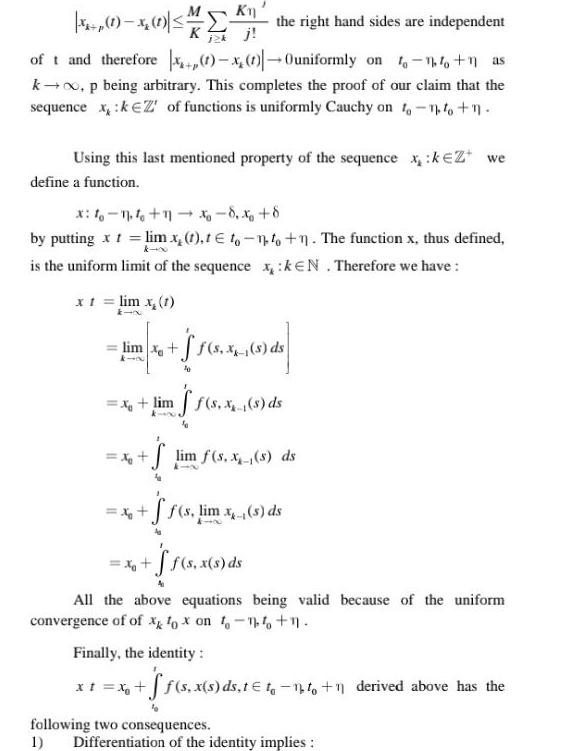Question:

# 1 x 1 the right hand sides are independent of t and

Last updated: 9/18/20231 x 1 the right hand sides are independent of t and therefore 1 1 Ouniformly on 1 1 1 as k p being arbitrary This completes the proof of our claim that the sequence x k of functions is uniformly Cauchy on to to n j2k Using this last mentioned property of the sequence xk Z we define a function x 10 11 10 11 8 8 by putting x t lim x t t to to n The function x thus defined k x is the uniform limit of the sequence x KEN Therefore we have x t lim x 1 lim x f s X s ds 61 10 x limff s x s ds S lim f s x s ds ff s lim x s ds x f s x s ds All the above equations being valid because of the uniform convergence of of x fox on to 14 1 Finally the identity x t x f f s x s ds t t 1 1 derived above has the following two consequences 1 Differentiation of the identity implies